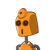# The 19th term of an AP is equal to three times its 6th term. If its 9th term is 19, find the A.P.​

The 19th term of an AP is equal to three times its 6th term. If its

9

th term is 19, find the A.P.​

### 1 thought on “The 19th term of an AP is equal to three times its 6th term. If its <br /><br />9<br /><br />th term is 19, find the A.P.​”

1.a19 = 3 × a6

a9 = 19

a19 = 3 × a6

a + 18d = 3 (a + 5d)

a + 18d = 3a + 15d

3a – a = 18d – 15d

2a = 3d

$$a = \frac{3d}{2}$$

$$a9 = 19 \\ a + 8d = 19 \\ \frac{3d}{2} + 8d = 19 \\ \\ \frac{3d + 16d}{2} = 19 \\ \\ 19d = 38 \\ d = \frac{38}{19} \\ \\ d = 2$$

$$a = \frac{3d}{2} \\ \\ a = \frac{3 \times 2}{2} \\ \\ a = \frac{6}{2} \\ \\ a = 3$$

$$a.p = 3 \: 5 \: 7…$$

## Verification :

$$a1 = a = 3 \\ d = 2 \\ a19 = a + 18d \\ a19 = 3 + 18 \times 2 \\ a19 = 3 + 36 \\ a19 = 39$$

$$a6 = a + 5d \\ a6 = 3 + 5 \times 2 \\ a6 = 3 + 10 \\ a6 = 13$$

$$a19 = 3 \times a6 \\ 39 = 3 \times 13 \\ 39 = 39 \\ hence \: verified$$